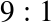# Odds

One way to represent a probability is with a number between 0 and 1, but that’s not the only way. If you have ever bet on a football game or a horse race, you have probably encountered another representation of probability, called odds.

You might have heard expressions like “the odds are three to one,” but you might not know what they mean. The odds in favor of an event are the ratio of the probability it will occur to the probability that it will not.

So if I think my team has a 75% chance of winning, I would say that the odds in their favor are three to one, because the chance of winning is three times the chance of losing.

You can write odds in decimal form, but it is most common to write them as a ratio of integers. So “three to one” is written.

When probabilities are low, it is more common to report the odds against rather than the odds in favor. For example, if I think my horse has a 10% chance of winning, I would say that the odds against are.

Probabilities and odds are different representations of the same information. Given a probability, you can compute the odds like this:

```def Odds(p):
return p / (1-p)```

Given the odds in favor, in decimal form, you can convert to probability like this:

```def Probability(o):
return o / (o+1)```

If you represent odds with ...

Get Think Bayes now with the O’Reilly learning platform.

O’Reilly members experience live online training, plus books, videos, and digital content from nearly 200 publishers.